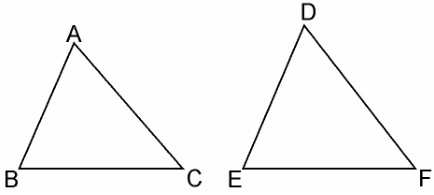Guru

# Sides of two similar triangles are in the ratio 4 : 9. Areas of these triangles are in the ratio (A) 2 : 3 (B) 4 : 9 (C) 81 : 16 (D) 16 : 81 Q.9

• 0

The important question of class 10th of chapter triangles of exercise 6.4, what is the simple method to solve this question Sides of two similar triangles are in the ratio 4 : 9. Areas of these triangles are in the ratio (A) 2 : 3 (B) 4 : 9 (C) 81 : 16 (D) 16 : 81

Share

1. Given, Sides of two similar triangles are in the ratio 4 : 9.Let ABC and DEF are two similar triangles, such that,

ΔABC ~ ΔDEF

And AB/DE = AC/DF = BC/EF = 4/9

As, the ratio of the areas of these triangles will be equal to the square of the ratio of the corresponding sides,

∴ Area(ΔABC)/Area(ΔDEF) = AB2/DE

∴ Area(ΔABC)/Area(ΔDEF) = (4/9)= 16/81 = 16:81

Hence, the correct answer is (D).

• 0